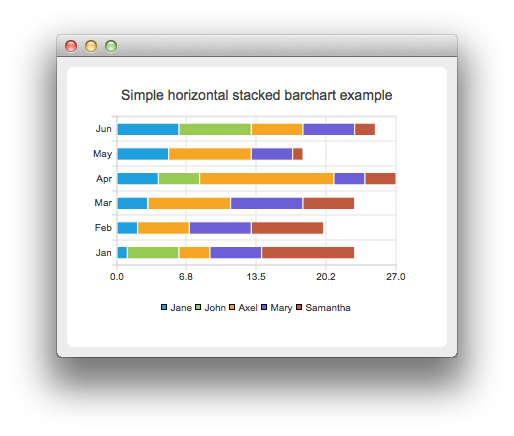# Creating Horizontal Stacked Bar Charts

Note: This is part of the Charts with Widgets Gallery example.

The stacked bar chart shows the data in sets as bars that are stacked on top of each other. The stacking is done per category. Creating a stacked horizontal bar chart is just like creating a regular bar chart, except that for a stacked horizontal bar chart, we use the QHorizontalStackedBarSeries api instead of QBarSeries.The bar sets are used in same way in all bar charts. To illustrate the difference between various barcharts, we use the same data in all examples. The data that barchart visualizes, is defined by the QBarSet instances. Here we create the sets and append data to them. The data is appended here with the << operator. Alternatively, the append method could also be used.

```auto set0 = new QBarSet("Jane");
auto set1 = new QBarSet("John");
auto set2 = new QBarSet("Axel");
auto set3 = new QBarSet("Mary");
auto set4 = new QBarSet("Samantha");

*set0 << 1 << 2 << 3 << 4 << 5 << 6;
*set1 << 5 << 0 << 0 << 4 << 0 << 7;
*set2 << 3 << 5 << 8 << 13 << 8 << 5;
*set3 << 5 << 6 << 7 << 3 << 4 << 5;
*set4 << 9 << 7 << 5 << 3 << 1 << 2;```

We create the series and append the bar sets to it. The series takes ownership of the bar sets. The series groups the data from sets to categories. The first values of each set are grouped together in the first category, the second values in the second category and so on.

```auto series = new QHorizontalStackedBarSeries;
series->append(set0);
series->append(set1);
series->append(set2);
series->append(set3);
series->append(set4);```

Here we create the chart object and add the series to it. We set the title for the chart with setTitle, and then turn on the animations of the series by calling setAnimationOptions(QChart::SeriesAnimations).

```auto chart = new QChart;
chart->setTitle("Simple Horizontal Stacked Bar Chart");
chart->setAnimationOptions(QChart::SeriesAnimations);```

To have the categories displayed on an axis, we need to create a QBarCategoryAxis first. Here we create a category axis with a list of categories and add it to the chart on the left-hand side, acting as the y-axis. The chart takes ownership of the axis. For x-axis we use a value axis, aligned to the bottom.

```QStringList categories {"Jan", "Feb", "Mar", "Apr", "May", "Jun"};
auto axisY = new QBarCategoryAxis;
axisY->append(categories);
series->attachAxis(axisY);
auto axisX = new QValueAxis;
```chart->legend()->setVisible(true);
`createDefaultChartView(chart);`